# RS Aggarwal Solutions for Class 10 Maths Chapter 1 Real Numbers Exercise 1C

R S Aggarwal Solutions for Class 10 Maths Chapter 1 Real Numbers, contains solutions for all Exercise 1C questions. Class 10 real numbers Exercise 1C based on the concept of rational numbers and their properties. All these questions are solved by subject experts using step by step problem-solving approach. R S Aggarwal Solutions of Class 10 and start practising.

### Download PDF of RS Aggarwal Solutions for Class 10 Maths Chapter 1 Real Numbers Exercise 1C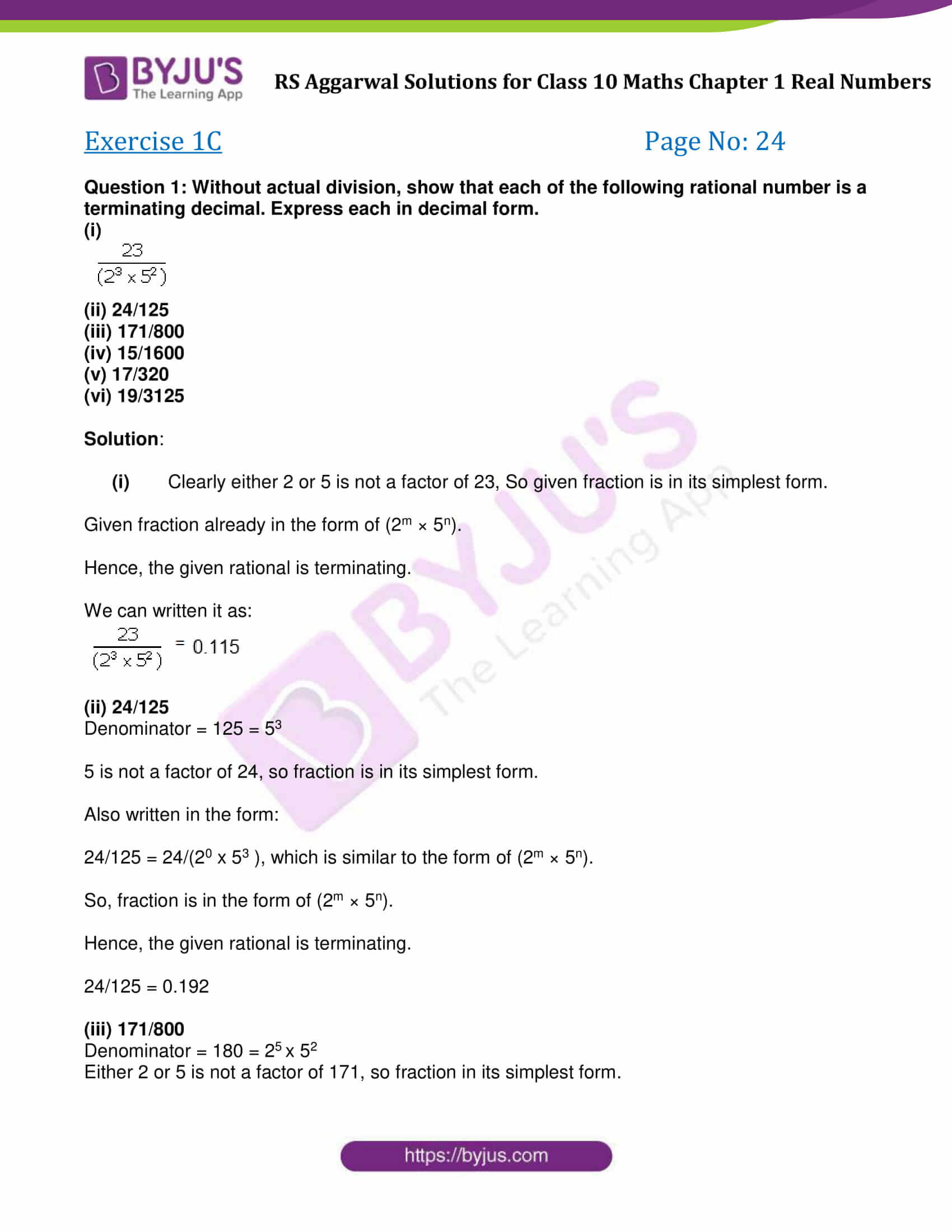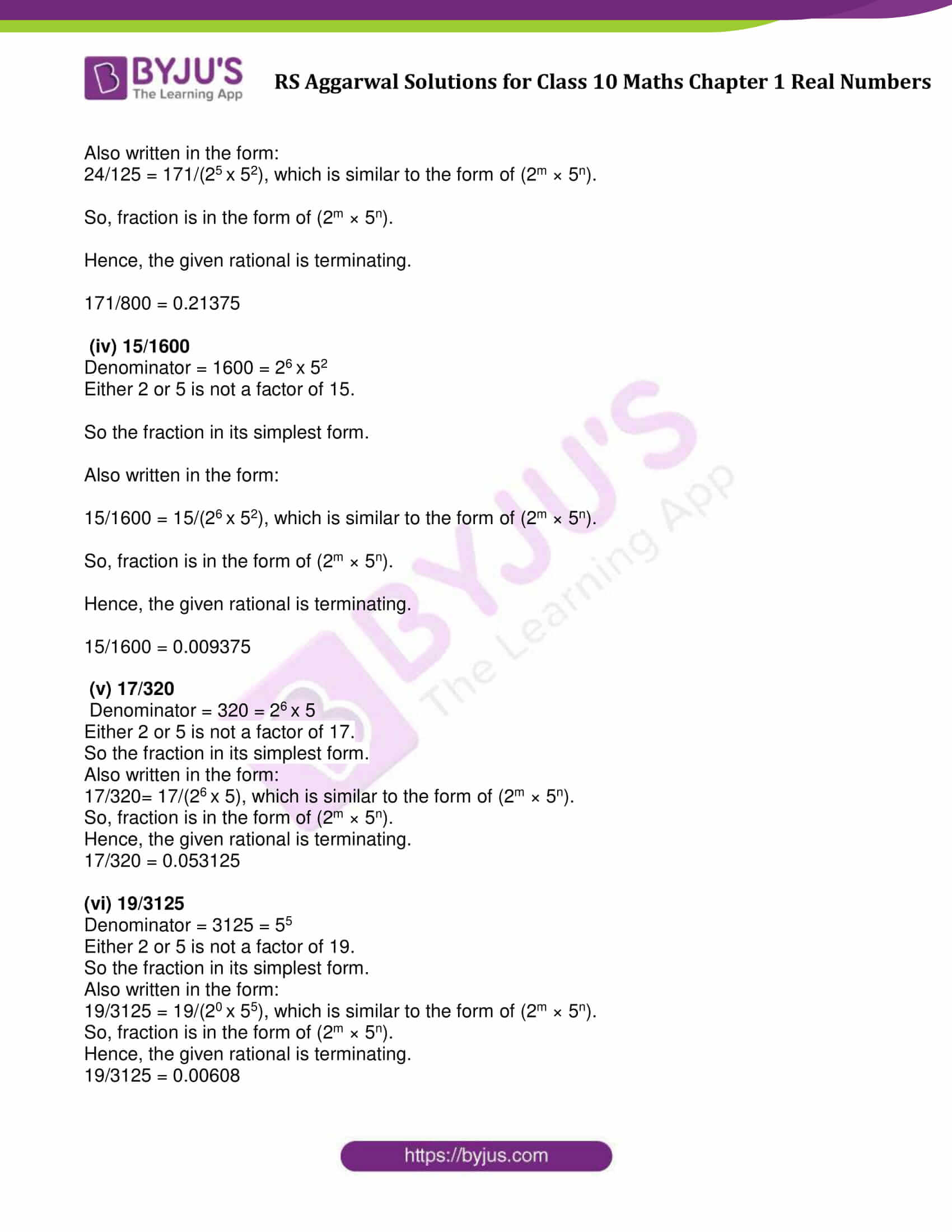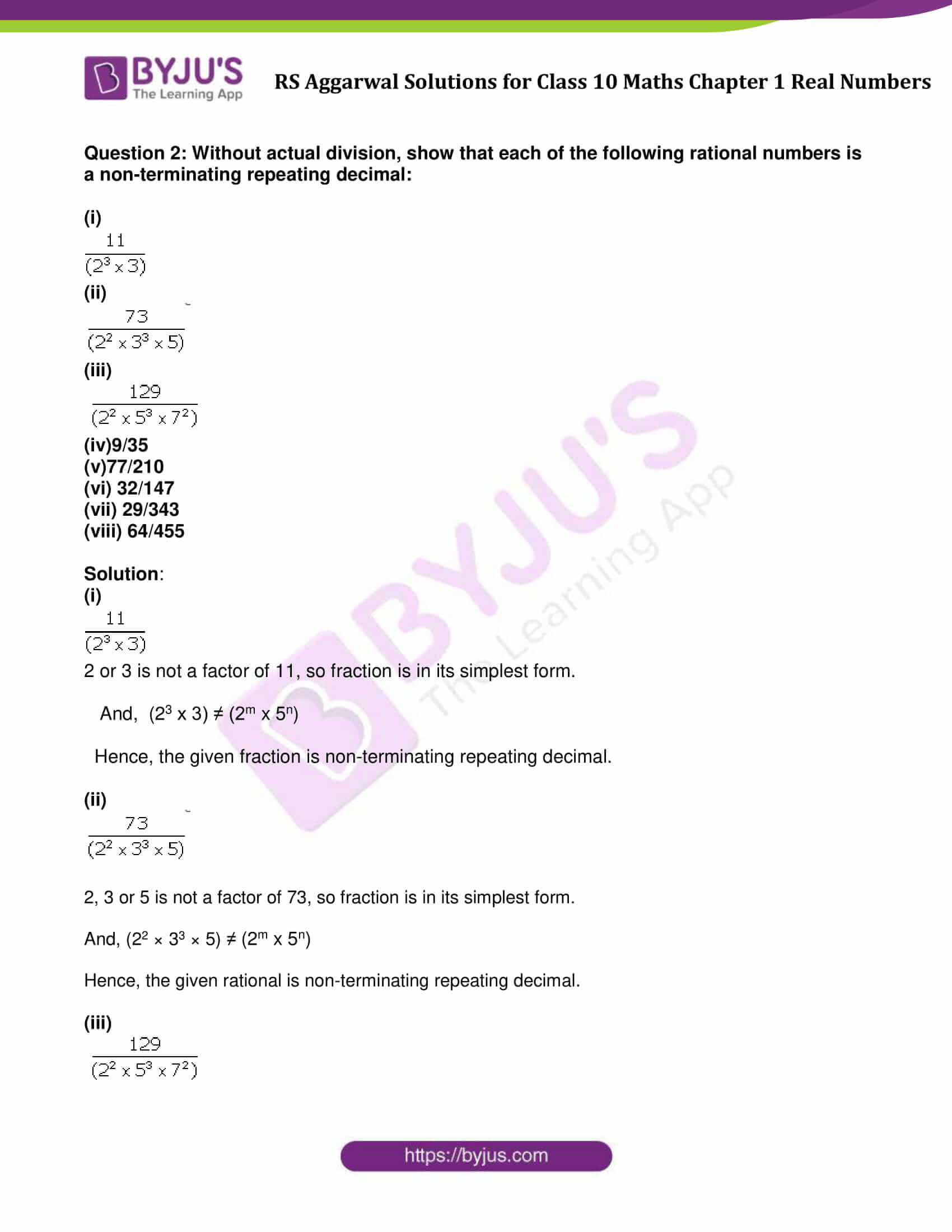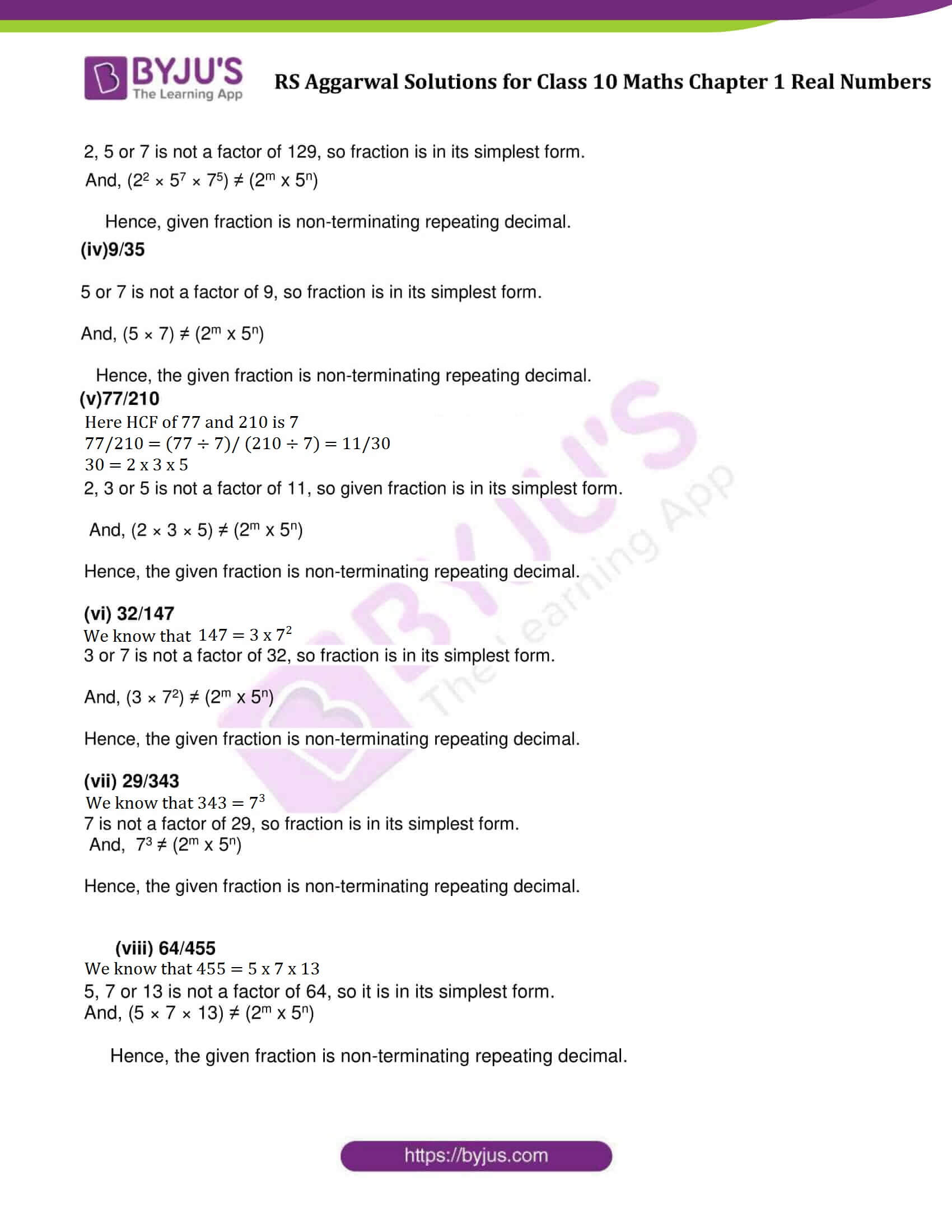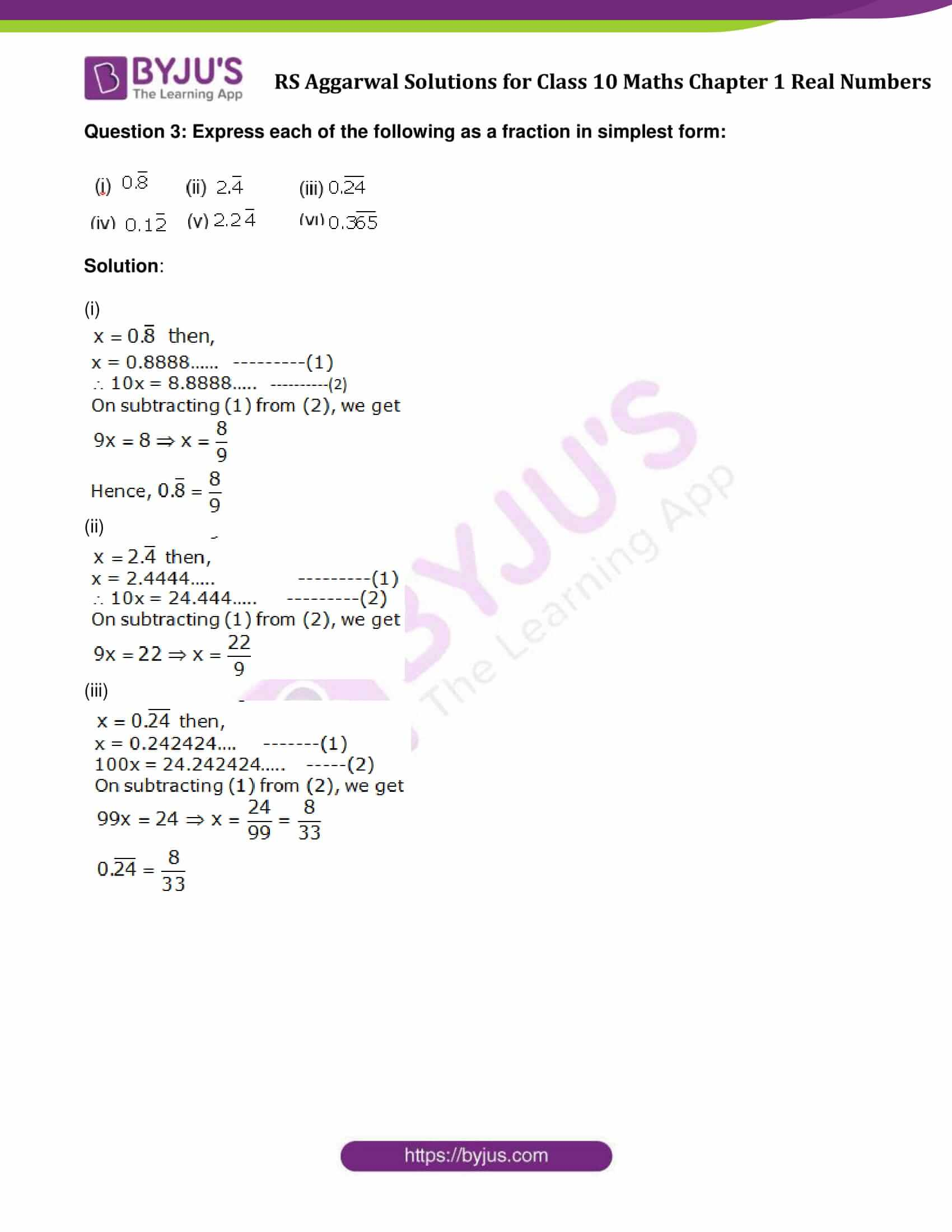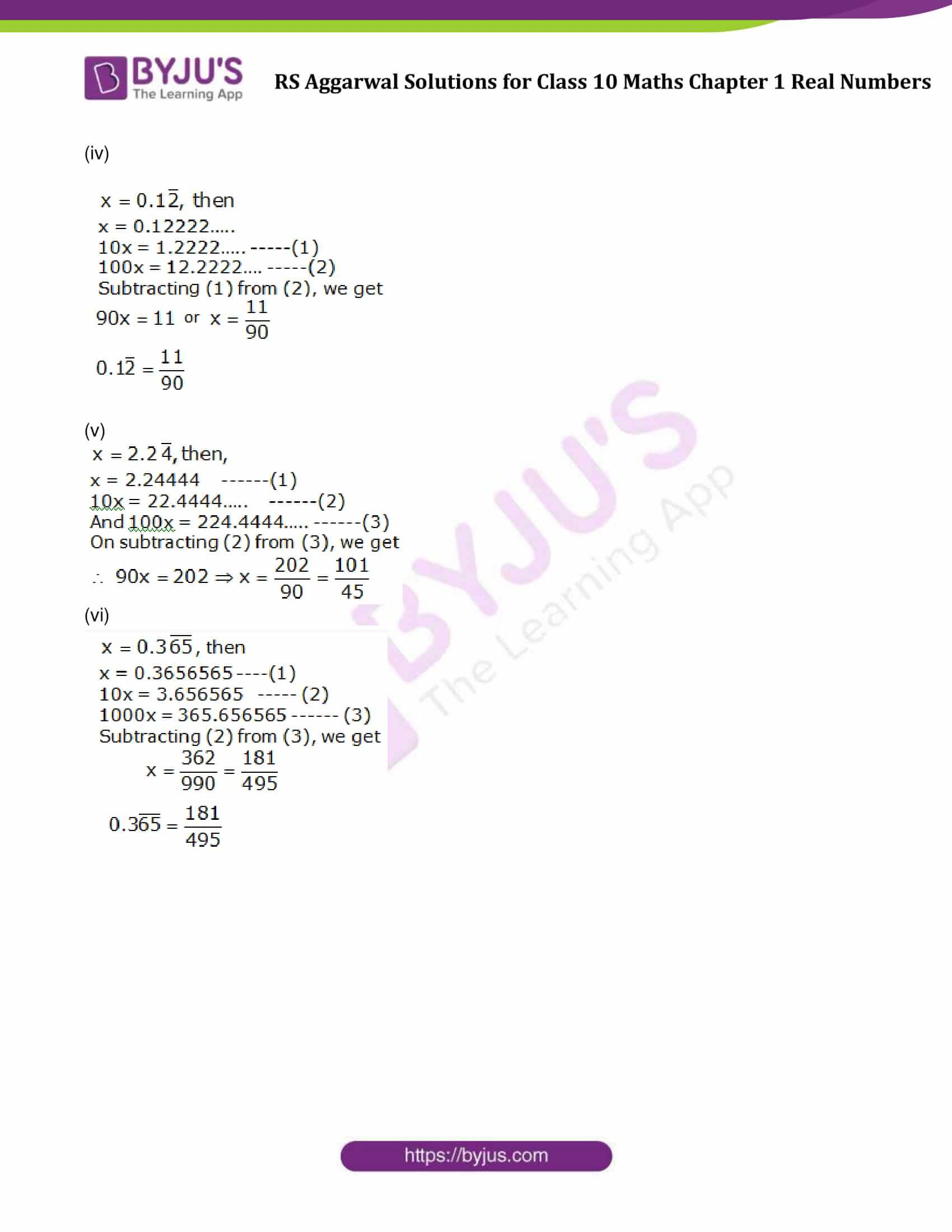### Access other exercise solutions of Class 10 Maths Chapter 1 Real Numbers

Exercise 1A Solutions: 10 Questions (Short Answers)

Exercise 1B Solutions: 27 Questions (Short Answers)

Exercise 1D Solutions: 11 Questions (Short Answers)

Exercise 1E Solutions: 23 Questions (Short Answers)

## Exercise 1C Page No: 24

Question 1: Without actual division, show that each of the following rational number is a terminating decimal. Express each in decimal form.

(i)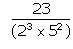(ii) 24/125

(iii) 171/800

(iv) 15/1600

(v) 17/320

(vi) 19/3125

Solution:Clearly either 2 or 5 is not a factor of 23, So given fraction is in its simplest form.

Given fraction already in the form of (2m × 5n).

Hence, the given rational is terminating.

We can written it as:

= 0.115

(ii) 24/125

Denominator = 125 = 53

5 is not a factor of 24, so fraction is in its simplest form.

Also written in the form:

24/125 = 24/(20 x 53 ), which is similar to the form of (2m × 5n).

So, fraction is in the form of (2m × 5n).

Hence, the given rational is terminating.

24/125 = 0.192

(iii) 171/800

Denominator = 180 = 25 x 52

Either 2 or 5 is not a factor of 171, so fraction in its simplest form.

Also written in the form:

24/125 = 171/(25 x 52), which is similar to the form of (2m × 5n).

So, fraction is in the form of (2m × 5n).

Hence, the given rational is terminating.

171/800 = 0.21375

(iv) 15/1600

Denominator = 1600 = 26 x 52

Either 2 or 5 is not a factor of 15.

So the fraction in its simplest form.

Also written in the form:

15/1600 = 15/(26 x 52), which is similar to the form of (2m × 5n).

So, fraction is in the form of (2m × 5n).

Hence, the given rational is terminating.

15/1600 = 0.009375

(v) 17/320

Denominator = 320 = 26 x 5

Either 2 or 5 is not a factor of 17.

So the fraction in its simplest form.

Also written in the form:

17/320= 17/(26 x 5), which is similar to the form of (2m × 5n).

So, fraction is in the form of (2m × 5n).

Hence, the given rational is terminating.

17/320 = 0.053125

(vi) 19/3125

Denominator = 3125 = 55

Either 2 or 5 is not a factor of 19.

So the fraction in its simplest form.

Also written in the form:

19/3125 = 19/(20 x 55), which is similar to the form of (2m × 5n).

So, fraction is in the form of (2m × 5n).

Hence, the given rational is terminating.

19/3125 = 0.00608

Question 2: Without actual division, show that each of the following rational numbers is a non-terminating repeating decimal:

(i)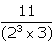(ii)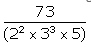(iii)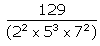(v)77/210
(vi) 32/147
(vii) 29/343
(viii) 64/455

Solution:

(i)2 or 3 is not a factor of 11, so fraction is in its simplest form.

And, (23 x 3) ≠ (2m x 5n)

Hence, the given fraction is non-terminating repeating decimal.

(ii)2, 3 or 5 is not a factor of 73, so fraction is in its simplest form.

And, (22 × 33 × 5) ≠ (2m x 5n)

Hence, the given rational is non-terminating repeating decimal.

(iii)2, 5 or 7 is not a factor of 129, so fraction is in its simplest form.

And, (22 × 57 × 75) ≠ (2m x 5n)

Hence, given fraction is non-terminating repeating decimal.

(iv)9/35

5 or 7 is not a factor of 9, so fraction is in its simplest form.

And, (5 × 7) ≠ (2m x 5n)

Hence, the given fraction is non-terminating repeating decimal.

(v)77/210

Here HCF of 77 and 210 is 7

77/210 = (77 ÷ 7)/ (210 ÷ 7) = 11/30

30 = 2 x 3 x 5

2, 3 or 5 is not a factor of 11, so given fraction is in its simplest form.

And, (2 × 3 × 5) ≠ (2m x 5n)

Hence, the given fraction is non-terminating repeating decimal.

(vi) 32/147

We know that 147 = 3 x 72

3 or 7 is not a factor of 32, so fraction is in its simplest form.

And, (3 × 72) ≠ (2m x 5n)

Hence, the given fraction is non-terminating repeating decimal.

(vii) 29/343

We know that 343 = 73

7 is not a factor of 29, so fraction is in its simplest form. And,  73 ≠ (2m x 5n)

Hence, the given fraction is non-terminating repeating decimal.

(viii) 64/455

We know that 455 = 5 x 7 x 13

5, 7 or 13 is not a factor of 64, so it is in its simplest form. And, (5 × 7 × 13) ≠ (2m x 5n)

Hence, the given fraction is non-terminating repeating decimal.

Question 3: Express each of the following as a fraction in simplest form: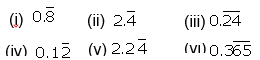Solution:

(i)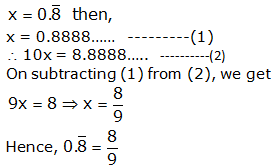(ii)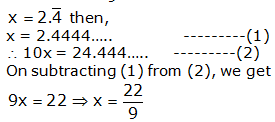(iii)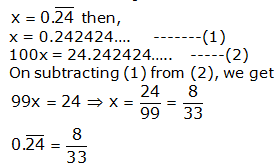(iv)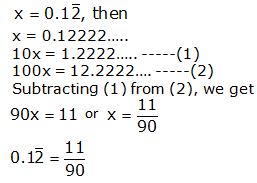(v)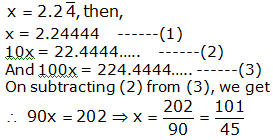(vi)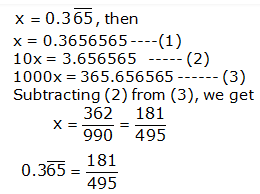## RS Aggarwal Solutions for Class 10 Maths Chapter 1 Real Numbers Exercise 1C

Class 10 Maths Chapter 1 Real Numbers Exercise 1C, based on the following topics and subtopics:

• Rational Numbers
• Some Important results on rational numbers